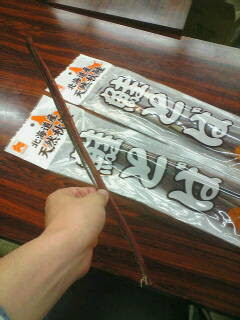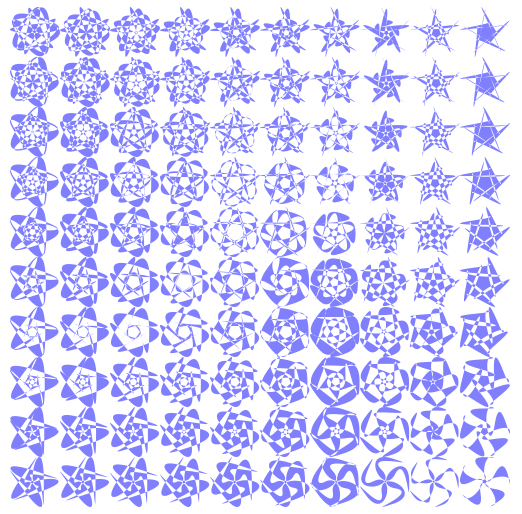## Monday, June 29, 2009

### SAKETOBA: Japanese redvines

Hi, it is NISHIO Hirokazu, today I'll write about a Japanese traditional food.Do you know Red Vines or Twizzlers? I know it is a popular candy in US, however it is not popular in Japan. Most of Japanese don't like its liquorice flavor. They say "It smells strange! It is too chewy! What's this lengthy food! Is it food?"

Today I realized SAKETOBA is such in Japan. It is very chewy. And it is made from salmons! I don't feel it smells strange, but I can imagine a lot of foreign people feel it strange. SAKETOBA is a salmon jerky. SAKE means salmon (you may know SAKE means alcoholic drink. The word has different accent.) and TOBA means swarm in Ainu; an ethnic group indigenous to northern part of Japan (Hokkaido). It is nice tidbits to go with drink.
Ivanhoe said... "I can tell you about some snack food that feels strange for a foreigner : dried squids ! Chewy and salted, it is not bad, but the first time I was shown it I wondered if I was really supposed to eat it..."

Yes, I love dried squids too!

## Tuesday, June 16, 2009

### How to calculate Bezier curves' bounding box

Hi, it is NISHIO Hirokazu. Today I wanted to make a lot of Bezier curves same size. And I got it.

Here is what I want to draw:To calculate bounding box of cubic Bezier seems easy, especially you know its parametric form. See Bézier curve - Wikipedia, the free encyclopedia

However, there are some pitfall. The derivative of Bezier equation is usually quadratic equation but not always. Solutions of the derivative may out of range, etc.

I publish following source code under MIT License. Feel free to use it.
`def calc_box(start, curves):    P0 = start    bounds = [[P0], [P0]]    for c in curves:        P1, P2, P3 = (            (c, c),             (c, c),             (c, c))        bounds.append(P3)        bounds.append(P3)        for i in [0, 1]:            f = lambda t: (                (1-t)**3 * P0[i]                 + 3 * (1-t)**2 * t * P1[i]                 + 3 * (1-t) * t**2 * P2[i]                + t**3 * P3[i])            b = 6 * P0[i] - 12 * P1[i] + 6 * P2[i]            a = -3 * P0[i] + 9 * P1[i] - 9 * P2[i] + 3 * P3[i]            c = 3 * P1[i] - 3 * P0[i]            if a == 0:                if b == 0:                    continue                t = -c / b                if 0 < t < 1:                     bounds[i].append(f(t))                continue            b2ac = b ** 2 - 4 * c * a            if b2ac < 0:                 continue            t1 = (-b + sqrt(b2ac))/(2 * a)            if 0 < t1 < 1: bounds[i].append(f(t1))            t2 = (-b - sqrt(b2ac))/(2 * a)            if 0 < t2 < 1: bounds[i].append(f(t2))        P0 = P3    x = min(bounds)    w = max(bounds) - x    y = min(bounds)    h = max(bounds) - y    return (x, y, w, h)`

Blaze Boy asked me about the structure, thank you. for each c in curves is 6-tuples: P1, P2, P3 = ((c, c), (c, c), (c, c)) P0 and P3 are terminal points of each bezier curves.

## Wednesday, June 3, 2009

### Fractal aurora(Koch curve)

Hi, it is NISHIO Hirokazu. I wondered how we see if there is aurora in Koch curve. So I wrote a python script run on Blender. Let see it(click to enlarge):The script is as follow. It is not beautifil because I'm just a beginner of Blender. A lot of lines are copy of sample.

`from Blender.Mathutils import Vectorfrom Blender import *from math import sin, cos, piclass Turtle(object):    def __init__(self):        self.x = 0.0        self.y = 0.0        self.dirx = 1.0        self.diry = 0.0        self.vert2d_list = [(0.0, 0.0)]        self.faces = []    def forward(self, mag):        self.x += self.dirx * mag         self.y += self.diry * mag         self.vert2d_list.append((self.x, self.y))    def rot(self, rad):        self.dirx, self.diry = (            self.dirx * cos(rad) - self.diry * sin(rad),            self.dirx * sin(rad) + self.diry * cos(rad))        def build_mesh(self, z=0.0, name="Mesh"):        verts3d = [Vector(x, y, z) for (x, y) in self.vert2d_list]        me = Mesh.New(name + str(z))          # create a new mesh        me.verts.extend(verts3d)          # add vertices to mesh        me.faces.extend(self.faces)           # add faces to the mesh (also adds edges)        return medef deg(x):    return 2 * pi / 360 * xdef makeHalo(x):    mat = Material.New('Halo')          # create a new Material called 'newMat'    mat.rgbCol = [0.7 * x, 0.7, 0.1]          # change its color    mat.setAlpha(0.3 * (1 - x))                     # mat.alpha = 0.2 -- almost transparent    #mat.emit = 0.7                        # equivalent to mat.setEmit(0.8)    #mat.mode |= Material.Modes.ZTRANSP    # turn on Z-Buffer transparency    #mat.setAdd(0.8)                       # make it glow    mat.setMode('Halo')                   # turn 'Halo' "on" and all others "off"    return matdef koch(level=1, unit=1):    if level == 0:        turtle.forward(unit)    else:        koch(level - 1, unit)        turtle.rot(deg(60))        koch(level - 1, unit)        turtle.rot(deg(-120))        koch(level - 1, unit)        turtle.rot(deg(60))        koch(level - 1, unit)        turtle = Turtle()koch(4, 0.6)for i in range(100):    ob = Object.New('Mesh', 'myObj')  # link mesh to an object    m = turtle.build_mesh(i / 5.0)    m.materials += [makeHalo(i / 100.0)]    ob.link(m)    sc = Scene.GetCurrent()          # link object to current scene    sc.link(ob)`